Next: MOSFETs Up: Semiconductor Devices Previous: MESFETs   Contents   Index

MESFET Models (both N and P Channel)

Type Names: nmf, pmf

The MESFET model is derived from the GaAs FET model of Statz et al. as described in. The dc characteristics are defined by the parameters vto, b, and beta, which determine the variation of drain current with gate voltage, alpha, which determines saturation voltage, and lambda, which determines the output conductance. The formula are given by

Id =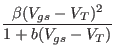1 -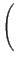1 -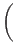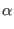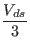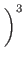(1 +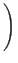Vds)    for    0 < Vds < 3/Id =(1 +Vds)    for    Vds > 3/Two ohmic resistances, rd and rs, are included. Charge storage is modeled by total gate charge as a function of gate-drain and gate-source voltages and is defined by the parameters cgs, cgd, and pb.

The parameters marked with an asterisk in the area column scale with the area parameter given in the device line.

 name MES Model Parameters area parameter units default example vto pinch-off voltage V -2.0 -2.0 beta * transconductance parameter A/V2 1.0e-4 1.0e-3 b * doping tail extending parameter 1/V 0.3 0.3 alpha * saturation voltage parameter 1/V 2 2 lambda channel length modulation parameter 1/V 0 1.0e-4 rd * drain ohmic resistance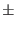0 100 rs * source ohmic resistance0 100 cgs * zero-bias G-S junction capacitance F 0 5pf cgd * zero-bias G-D junction capacitance F 0 1pf pb gate junction potential V 1 0.6 kf flicker noise coefficient - 0 - af flicker noise exponent - 1 - fc coefficient for forward-bias depletion capacitance formula - 0.5 -Next: MOSFETs Up: Semiconductor Devices Previous: MESFETs   Contents   Index
Stephen R. Whiteley 2019-06-03